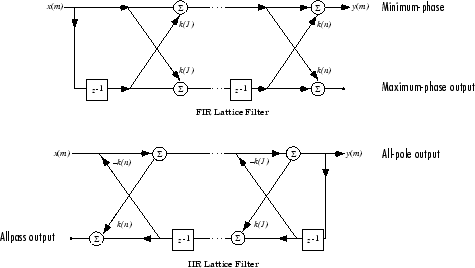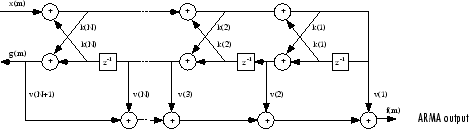## 离散时间系统模型

### 传递函数

`$Y\left(z\right)=\frac{b\left(1\right)+b\left(2\right){z}^{-1}+\dots +b\left(n+1\right){z}^{-n}}{a\left(1\right)+a\left(2\right){z}^{-1}+\dots +a\left(m+1\right){z}^{-m}}X\left(z\right).$`

### 零极点增益

`$H\left(z\right)=\frac{q\left(z\right)}{p\left(z\right)}=k\frac{\left(z-q\left(1\right)\right)\left(z-q\left(2\right)\right)...\left(z-q\left(n\right)\right)}{\left(z-p\left(1\right)\right)\left(z-p\left(2\right)\right)...\left(z-p\left(n\right)\right)}.$`

`poly``roots` 函数在多项式表示和零极点增益表示之间转换。例如，一个简单 IIR 滤波器如下

```b = [2 3 4]; a = [1 3 3 1]; ```

```q = roots(b) p = roots(a) % Gain factor k = b(1)/a(1) ```

```bb = k*poly(q) aa = poly(p) ```

`$H\left(z\right)=\frac{2+3{z}^{-1}+4{z}^{-2}}{1+3{z}^{-1}+3{z}^{-2}+{z}^{-3}}=\frac{z\text{\hspace{0.17em}}\left(2{z}^{2}+3z+4\right)}{{z}^{3}+3{z}^{2}+3z+1}.$`

### 状态空间

`$\begin{array}{c}x\left(n+1\right)=Ax\left(n\right)+Bu\left(n\right)\\ y\left(n\right)\text{ }\text{ }\text{ }\text{ }\text{ }\text{ }\text{ }\text{ }\text{ }\text{ }\text{ }\text{ }\text{ }\text{ }\text{ }=Cx\left(n\right)+Du\left(n\right),\end{array}$`

### 部分分式展开式（残差形式）

`$\frac{b\left(z\right)}{a\left(z\right)}=\frac{r\left(1\right)}{1-p\left(1\right){z}^{-1}}+...+\frac{r\left(n\right)}{1-p\left(n\right){z}^{-1}}+k\left(1\right)+k\left(2\right){z}^{-1}+...+k\left(m-n+1\right){z}^{-\left(m-n\right)}$`

`$\frac{r\left(j\right)}{1-p\left(j\right){z}^{-1}}+\frac{r\left(j+1\right)}{{\left(1-p\left(j\right){z}^{-1}\right)}^{2}}...+\frac{r\left(j+{s}_{r}-1\right)}{{\left(1-p\left(j\right){z}^{-1}\right)}^{{s}_{r}}}$`

Signal Processing Toolbox™ 中的 `residuez` 函数用于将传递函数转换为部分分式展开式形式，或者反之。`residuez` 末尾的“`z`”表示 z 域或离散域。`residuez` 以列向量 `p` 形式返回极点，以列向量 `r` 形式返回极点对应的残差，以行向量 `k` 形式返回原始传递函数的任何不恰当部分。如果两个极点的差值的量级小于任一极点的量级的 0.1%，则 `residuez` 确定这两个极点相同。

`$H\left(z\right)=\frac{-4+8{z}^{-1}}{1+6{z}^{-1}+8{z}^{-2}}$`

```b = [-4 8]; a = [1 6 8]; [r,p,k] = residuez(b,a) ```

`$H\left(z\right)=\frac{-12}{1+4{z}^{-1}}+\frac{8}{1+2{z}^{-1}}$`

`$h\left(n\right)=-12{\left(-4\right)}^{n}+8{\left(-2\right)}^{n},\text{ }n=0,1,2,\dots$`

```imp = [1 0 0 0 0]; resptf = filter(b,a,imp) respres = filter(r(1),[1 -p(1)],imp)+... filter(r(2),[1 -p(2)],imp) ```

### 二阶节 (SOS)

`$H\left(z\right)=\prod _{k=1}^{L}{H}_{k}\left(z\right)=\prod _{k=1}^{L}\frac{{b}_{0k}+{b}_{1k}{z}^{-1}+{b}_{2k}{z}^{-2}}{{a}_{0k}+{a}_{1k}{z}^{-1}+{a}_{2k}{z}^{-2}}$`

`$\text{sos}=\left(\begin{array}{llllll}{b}_{01}\hfill & {b}_{11}\hfill & {b}_{21}\hfill & {a}_{01}\hfill & {a}_{11}\hfill & {a}_{21}\hfill \\ {b}_{02}\hfill & {b}_{12}\hfill & {b}_{22}\hfill & {a}_{02}\hfill & {a}_{12}\hfill & {a}_{22}\hfill \\ ⋮\hfill & ⋮\hfill & ⋮\hfill & ⋮\hfill & ⋮\hfill & ⋮\hfill \\ {b}_{0L}\hfill & {b}_{1L}\hfill & {b}_{2L}\hfill & {a}_{0L}\hfill & {a}_{1L}\hfill & {a}_{2L}\hfill \end{array}\right)$`

### 格型结构体

FIR 和 IIR 格型滤波器结构图```b = [1.0000 0.6149 0.9899 0.0000 0.0031 -0.0082]; ```

```k = tf2latc(b) ```

```a = [1 1/2 1/3]; ```

`[k,v] = tf2latc(b,a); `

```b = latc2tf(k); ```

### 卷积矩阵

```b = [1 2 3]; x = randn(3,1); C = convmtx(b',3); ```

`b``x` 的卷积的两种等效方法如下。

```y1 = C*x; y2 = conv(b,x); ```